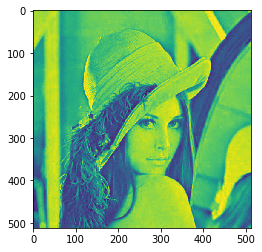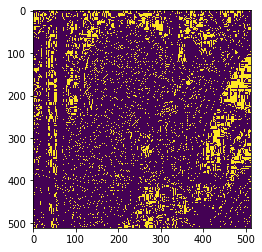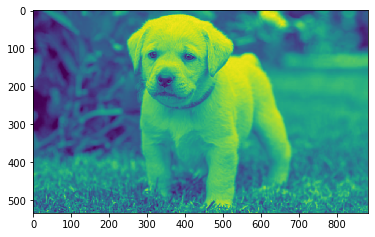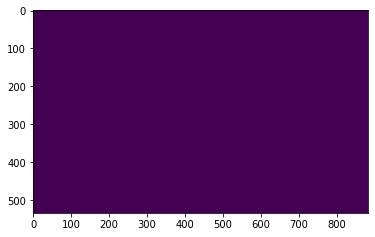GFG App
Open AppBrowser
Continue

# Mahotas – Local Maxima in Image

In this article we will see how we can find local maxima of the image in mahotas. Local maxima is basically local peaks in the image. In this tutorial we will use “lena” image, below is the command to load it.

`mahotas.demos.load('lena')`

Below is the lena imageIn order to do this we will use mahotas.locmax method
Syntax : mahotas.locmax(img)
Argument : It takes image object as argument
Return : It returns image object

Note : Input image should be filtered or should be loaded as grey
In order to filter the image we will take the image object which is numpy.ndarray and filter it with the help of indexing, below is the command to do this

`image = image[:, :, 0]`

Below is the implementation

## Python3

 `# importing required libraries` `import` `mahotas` `import` `mahotas.demos` `from` `pylab ``import` `gray, imshow, show` `import` `numpy as np` `import` `matplotlib.pyplot as plt` `  `  `# loading image` `img ``=` `mahotas.demos.load(``'lena'``)`     `  `  `# filtering image` `img ``=` `img.``max``(``2``)`   `print``(``"Image"``)` `  `  `# showing image` `imshow(img)` `show()`   `# getting local maxima of the image` `new_img ``=` `mahotas.locmax(img)` ` `    `# showing image` `print``(``"Local Maxima"``)` `imshow(new_img)` `show()`

Output :

`Image``Local Maxima`Another example

## Python3

 `# importing required libraries` `import` `mahotas` `import` `numpy as np` `from` `pylab ``import` `gray, imshow, show` `import` `os` `import` `matplotlib.pyplot as plt` ` `  `# loading image` `img ``=` `mahotas.imread(``'dog_image.png'``)`     `# filtering image` `img ``=` `img[:, :, ``0``]` `  `  `print``(``"Image"``)` `  `  `# showing image` `imshow(img)` `show()`   `# getting local maxima of the image` `new_img ``=` `mahotas.locmax(img)` ` `    `# showing image` `print``(``"Local Maxima"``)` `imshow(new_img)` `show()`

Output :

`Image``Local Maxima`My Personal Notes arrow_drop_up